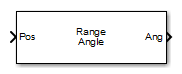# Range Angle Calculator

Range and angle calculations

## Library

Environment and Target

`phasedenvlib`

•## Description

The Range Angle Calculator block calculates the ranges and/or the azimuth and elevation angles of several positions with respect to a reference position and with respect to a reference axes orientation. The reference position and reference axes can be specified in the block dialog or using input ports.

## Parameters

Propagation model

Specify the propagation model by setting this parameter to ```Free space``` or `Two-ray`.

Reference position source

Specify the reference position source by setting this parameter to `Property` or `Input port`. If Reference position source is set to `Property`, set the position using the Reference position parameter. If Reference position source is set to ```Input port```, use the input port labeled `RefPos`.

Reference position

Specify the reference position as a 3-by-1 vector of rectangular coordinates in meters in the form `[x;y;z]`. The reference position serves as the origin of the local coordinate system. Ranges and angles of the input positions are measured with respect to the reference position. This parameter appears only when Reference position source is set to `Property`.

Reference axes source

Specify the reference axes source by setting this parameter to `Property` or `Input port`. If Reference axes source is set to `Property`, set the axes using the Reference axes parameter. If Reference axes source is set to ```Input port```, use the input port labeled `RefAxes`.

Reference axes

Specify the reference axes of the local coordinate system with which to calculate range and angles in the form of a 3-by-3 orthonormal matrix. Each column of the matrix specifies the direction of an axis for the local coordinate system in the form of `[x; y; z]` with origin at the reference position. This parameter appears only when Reference axes source is set to `Property`.

Output(s)

Specify the desired output(s) of the block. Each type of output is sent to a different port depending on the parameter value.

ValuePort
`Angle``Ang`
`Range``Range`
`Range and Angle``Ang` and `Range`
Simulate using

Block simulation method, specified as `Interpreted Execution` or ```Code Generation```. If you want your block to use the MATLAB® interpreter, choose `Interpreted Execution`. If you want your block to run as compiled code, choose `Code Generation`. Compiled code requires time to compile but usually runs faster.

Interpreted execution is useful when you are developing and tuning a model. The block runs the underlying System object™ in MATLAB. You can change and execute your model quickly. When you are satisfied with your results, you can then run the block using ```Code Generation```. Long simulations run faster than they would in interpreted execution. You can run repeated executions without recompiling. However, if you change any block parameters, then the block automatically recompiles before execution.

When setting this parameter, you must take into account the overall model simulation mode. The table shows how the Simulate using parameter interacts with the overall simulation mode.

When the Simulink® model is in `Accelerator` mode, the block mode specified using Simulate using overrides the simulation mode.

Acceleration Modes

 Block Simulation Simulation Behavior `Normal` `Accelerator` `Rapid Accelerator` `Interpreted Execution` The block executes using the MATLAB interpreter. The block executes using the MATLAB interpreter. Creates a standalone executable from the model. `Code Generation` The block is compiled. All blocks in the model are compiled.

## Ports

Note

The block input and output ports correspond to the input and output parameters described in the `step` method of the underlying System object. See link at the bottom of this page.

PortSupported Data Types
`Pos`Double-precision floating point
`RefPos`Double-precision floating point
`RefAxes`Double-precision floating point
`Range`Double-precision floating point
`Ang`Double-precision floating point